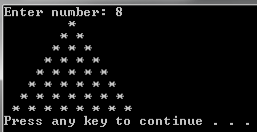LearnFast.Ninja

# C Sharp program to print asterisk isosceles triangle

First we asking the user to enter some number, this will be the size of the triangle. Then we call the Draw_Isosceles_Triangle function and send as parameter the users input. Now we need to implement the Draw_Isosceles_Triangle function, We Run all over the triangle with the first for loop. The first inner loop will draw some sapces before we start drawing the asterisks sequence. The second inner loop will draw asterisks and one space because we want a isosceles triangle. before the next iteration we create new line for the next asterisks sequence. Copy the working code example and run the program to see the result for yourself.

Working code example:

using System;

class Program

{

static void Main(string[] args)

{

int input = 0;

try

{

Console.Write("Enter number: ");

}

catch (Exception e)

{

Console.WriteLine(e.Message);

}

Draw_Isosceles_Triangle(input);

}

public static void Draw_Isosceles_Triangle(int num)

{

// Run all over the triangle

for (int i = 0; i < num; i++)

{

// Draw some sapces before we start drawing the asterisks sequence

for (int j = num - i; j > 0; j--)

Console.Write(" ");

// Draw asterisks and one space because we want a isosceles triangle

for (int j = 0; j <= i; j++)

Console.Write("*" + " ");

// Create new line for the next asterisks sequence

Console.WriteLine();

}

}

}// end Program class

Result:Tags

c-sharp